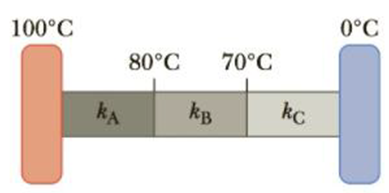Chapter 11, Problem 11CQ

Chapter
Section
Textbook Problem

Figure CQ11.11 shows a composite bar made of three different materials that connects a hot reservoir at 100°C to a cold reservoir at 0°C. If the sections A, B, and C all have the same dimensions and the temperatures shown in the figure are constant, rank the thermal conductivities from largest to smallest.Figure CQ11.11

To determine
Ranking of thermal conductivities from largest to smallest.

Explanation

Every substance has difference thermal conductivity. The substance with larger thermal conductivity conducts heat quicker than the substance with smaller thermal conductivity.

The rate of energy transfer by conduction through a rod area A and thickness L is,

P=kAΔTL , ΔT is the difference in temperature between the ends of the rod.

In the Figure CQ11.11, the sections A, B and C all have same dimensions with difference thermal conductivity. So, the larger difference in temperature between the end point denotes larger thermal conductivity and smaller difference in temperature between the end point denotes smaller thermal conductivity.

Section A

ΔTA=(100°80°)=20°

Section B</

Still sussing out bartleby?

Check out a sample textbook solution.

See a sample solution

The Solution to Your Study Problems

Bartleby provides explanations to thousands of textbook problems written by our experts, many with advanced degrees!

Get Started

Today, an estimated 69 percent of the adults in the United States are overweight or obese.

Nutrition: Concepts and Controversies - Standalone book (MindTap Course List)

What is the function of the central vacuole in plants?

Biology: The Dynamic Science (MindTap Course List)

Match the term listed in Column A with its definition from Column B

Nutrition Through the Life Cycle (MindTap Course List)